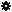﻿ Object Model: Edge.GrowNeighborEdges

Edge.GrowNeighborEdges

## Edge.GrowNeighborEdgesv3.0

### Description

Returns a collection of Edge objects containing all edges that are adjacent to each point in this edge, within a given distance.

### C# Syntax

 ```EdgeCollection Edge.GrowNeighborEdges( Int32 in_lDistance ); ```

### Scripting Syntax

 ```oReturn = Edge.GrowNeighborEdges( [Distance] ); ```

### Parameters

Parameter Type Description
Distance Integer Distance is an integer value representing the degree of neighborhood (for example, degree=2 for an edge means its adjacent edges plus the adjacent edges of the adjacent edges).

Default Value: 1

### Examples

#### VBScript Example

 ```set oCube = ActiveSceneRoot.AddGeometry( "Cube", "MeshSurface" ) set oGeometry = oCube.ActivePrimitive.Geometry for each Edge in oGeometry.Edges set oNeighbors = Edge.GrowNeighborEdges str = "Edges around Edge(" & Edge.index & ") are :" for each n in oNeighbors str = str & " " & n.index next logmessage str next ```